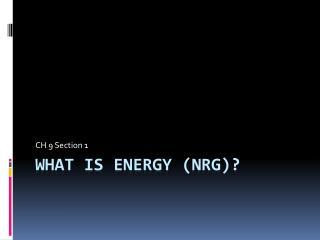DownloadDownload PresentationWhat is Energy ( nrg )?

# What is Energy ( nrg )?

Download Presentation## What is Energy ( nrg )?

- - - - - - - - - - - - - - - - - - - - - - - - - - - E N D - - - - - - - - - - - - - - - - - - - - - - - - - - -
##### Presentation Transcript

1. CH 9 Section 1 What is Energy (nrg)?

2. NRG: The ability to do work. • Work occurs when a force causes an object to move in the direction of the force. • Example: Playing tennis • Tennis player does work on the racket • Racket does work on the ball • Ball does work on the net • Each time work is done, something is given by one object to another that allows it to do work. • That something is energy. Work is a xfer of NRG.

3. Kinetic NRG: NRG of motion. • All moving objects have kinetic NRG. • Example: Biking • Getting on the bike • Peddling • Pushing the brake • Standing still is Potential, except that the heart, lungs, eyes, blood are all moving • internally.

4. Objects with KE can do work. • Bowling • Throw the ball down the lane….work is done using kinetic NRG. • Ball then does work on the pins.

5. KE Depends on SPEED and MASS • The faster something moves, the more KE it has. • Speed has a greater effect on KE than mass does. • KE=mv2 2 • V = velocity • The more massive the object, the more KE it has. • In the KE equation, m stands for mass.

6. Scenario: If a small car, an SUV and a semi-truck are all going the same speed, which one has the most kinetic NRG? The semi-truck has the most KE because it has the Most mass and has the same speed as the other Objects.

7. Potential NRG = NRG of Position. Bow and arrow: Bow is not moving, but has NRG because work has been done to change its shape. Stretched rubber band Elastic Potential NRG High dive vs. Low dive • Weight and height affect gravitational potential energy. • At the same height, the diver with more mass has more PE. • At two different heights, the diver at the higher point has more PE. • Gravitational PE=weight x height

8. Mechanical NRG = potential NRG + kinetic NRG Mechanical NRG: Total NRG of motion + position of an object.

9. When one goes up, the other must come down. • The total amount of mechanical energy is merely the sum of these two forms of energy. And finally, an object with mechanical energy is able to do work on another object. • http://www.glenbrook.k12.il.us/gbssci/phys/Class/energy/u5l1d.html

10. Review: • How are work and NRG related? • Energy is the ability to do work; work is a xfer of NRG. • What is the difference between kinetic and potential NRG? 2. KE is the energy of motion; PE is an objects NRG due to its position or shape. 3. Explain why a high speed collision may cause more damage than the same objects traveling at a low speed. 3. The vehicles would have more KE due to their high speed. The vehicles would do more work on each other, resulting in large amounts of damage.